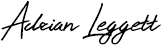## How to convert Maidenhead Locator to Latitude and Longitude

This post shows the step by step process of decoding a Maidenhead locator code to calculate the latitude and longitude at the centre of a square. I have used this calculation in logging software I have been continuously developing the last three years.

Maidenhead locator codes are used to identify the operators location and are often referred to as a QTH locator, grid locator or grid square. Besides other things they and are used in contesting not only a means to identify the location but also to calculate contest points.

Over the last three years I’ve been continuously developing my logging software to record my amateur radio contacts. I initially wrote the program to log general contacts but recently enhanced to include contest logging features.

A requirement of my software is to calculate the distance between the centre of the Maidenhead locator of my QTH and the centre of the remote operators Maidenhead locator code.

I’ve written this post because despite finding lots of computer programs and apps that automatically do it for you, people posting code of computer programs written to do it and information that is complicated to understand, I could not find anywhere where the process is easily explained. There is nothing complex in this; just basic maths. So apart from a calculator (or not) the only other thing you will need to do the decode is an ASCII character set chart, which I have added a link to below.

Firstly you need to understand a few key things about the locator:

• The first pair of characters represent latitude and longitude ( ‘I‘ = longitude, ‘O‘ = latitude).
• The pair of numbers ‘93‘ represent a square. Each  square represents 1° of latitude by 2° of longitude.
• The second pair of letters ‘ob‘ represent a sub-square. They are mostly (but not always) lower case. Each pair of letters represents of 2.5′ of latitude by 5′ of longitude.

So how do you convert a Maidenhead locator code of IO93ob? Lets go!

## ASCII CHARACHTER SET

Firstly you’ll need an ASCII character code set. Click here to open.

## DECODE LATITUDE

There are four steps (1, 2, 3 and 4) required to decode the latitude locator code IO93ob:

Step 1:

1a) Find the ASCII charachter code for the 2nd character of the locator code

O  =  79

1b) Deduct 65 from 79

79 – 65 = 14

1c) Multiply by 10

14 x 10 = 140

Step 2:

2a) Get number from position 4

3

Step 3:

3a) Find the ASCII charachter code for the 6th character of the locator code

b = 98

3b) Deduct 97 from 98

98 – 97 = 1

3c) Divide by 24

1 / 24 = 0.041667

0.041667 + (1 / 48)) = 0.0625

3e) Deduct 90

0.0625 – 90 = -89.9375

Step 4:

Latitude = [result from step 1] + [result from step 2] + [result from step 3]

Latitude = 140 + 3 + -89.9375

Latitude = 53.0625

## DECODE LONGITUDE

There are four steps (1, 2, 3 and 4) required to decode locator code IO93ob:

Step 1:

1a) Find the ASCII charachter code for the 1st character of the locator code

I  =  73

1b) Deduct 65 from 73

73 – 65 = 8

1c) Multiply by 20

14 x 10 = 160

Step 2:

2a) Get number from position 3

9

2b) Multiply by 2

9 x 2 = 18

Step 3:

3a) Find the ASCII charachter code for the 5th character of the locator code

o = 111

3b) Deduct 97 from 111

111 – 97 = 14

3c) Divide by 12

14 / 12 = 1.1666

1.1666 + (1 / 24)) = 1.20826

Step 4:

4a) Add results A, B and C then deduct 180

(160 + 18 + 1.20826) – 180 = -0.792

Latitude = -0.792

The latitude and longitude at the centre point of Maidenhead locator square is 53.0625-0.792

## TESTING

I plotted Maidenhead locator square IO93ob on the map (red square) and then plotted latitude and longitude 53.0625-0.792 (red dot). As you can see this is dead centre of the square.And it is as simple as that! In another post I will be showing how to calculate the distance between the centre of two different locator codes using latitudes and longitudes calculated using the above process.

Why not take a look at my YouTube channel here or Twitter posts here.

You can also register for my website here.

Thanks

73 de M0NWK## Multi-sensor real-time temperature monitoring and logging system using Arduino

### 1 comment

1.Gregory Smith says:

Very well explained. Do you know any online tool that convert Maidenhead locator to Lati Long coordinates? Something like this website that converts between Lati Long formats https://latitude-longitude.net . It was great if there was a similar website where we could simply put the Maidenhead locator code and the map show its location and GPS coordinates.